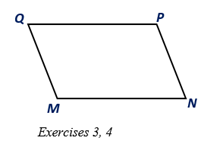Chapter 4.1, Problem 3E### Elementary Geometry for College St...

6th Edition
Daniel C. Alexander + 1 other
ISBN: 9781285195698

#### Solutions

Chapter
Section### Elementary Geometry for College St...

6th Edition
Daniel C. Alexander + 1 other
ISBN: 9781285195698
Textbook Problem
52 views

# MNPQ is a parallelogram. Suppose that M Q = 5 , M N = 8 , and m∠M = 110 0 . Find: a) QP c) m∠Q b) NP d) m∠PTo determine

a.

To find:

The length of QP in the parallelogram MNPQ.

Explanation

Given:

MNPQ is a parallelogram and suppose that MQ=5, MN=8, and m∠M=110°.

Corollary:

The opposite sides of a parallelogram are congruent.

Approach:

Here the sides QP-</

To determine

b.

To find:

The length of NP in the parallelogram MNPQ.

To determine

c.

To find:

The measures of m∠Q in a parallelogram MNPQ.

To determine

d.

To find:

The measure of angle m∠P in the parallelogram MNPQ.

### Still sussing out bartleby?

Check out a sample textbook solution.

See a sample solution

#### The Solution to Your Study Problems

Bartleby provides explanations to thousands of textbook problems written by our experts, many with advanced degrees!

Get Started

#### Find more solutions based on key concepts